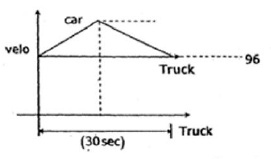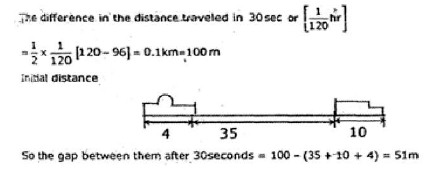Courses

# Engineering Mechanics

## 15 Questions MCQ Test GATE Mechanical (ME) 2022 Mock Test Series | Engineering Mechanics

Description
This mock test of Engineering Mechanics for GATE helps you for every GATE entrance exam. This contains 15 Multiple Choice Questions for GATE Engineering Mechanics (mcq) to study with solutions a complete question bank. The solved questions answers in this Engineering Mechanics quiz give you a good mix of easy questions and tough questions. GATE students definitely take this Engineering Mechanics exercise for a better result in the exam. You can find other Engineering Mechanics extra questions, long questions & short questions for GATE on EduRev as well by searching above.
QUESTION: 1

### Directions: Q. 1 - 5  carry 1 mark each A constant force of 10 N is acting on a body of mass ‘m’ for 10 seconds and changes its velocity from 10m/s to 15m/s. Calculate the mass of the body

Solution: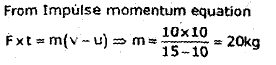QUESTION: 2

### A solid cylinder of diameter 5.0 m has a height of 6.0 m. Find the meta-centric height of the cylinder if the specific gravity of the material of cylinder 0.45 and it is floating in water with its axis vertical. State whether the equilibrium is stable or unstable.

Solution:

BG=Centre of pontoon – Centre of immersed portion=0.3-0.45*0.3=1.65
Metacentric height=I/∀ -BG
I=π*r⁴=π*2.5⁴
∀=π*r*r*h=π*2.5*2.5*6
Metacentric height=-0.61.

QUESTION: 3

### The ratio of limiting friction and normal reaction is known as

Solution:
QUESTION: 4

If Fx and Fy are algebraic sum of horizontal forces and vertical forces at a point under equilibrium condition, then angle made by resultant force with horizontal direction is

Solution:
QUESTION: 5

If three forces acting in one plane upon a rigid body, keeping it in equilibrium, then they must either

Solution:
QUESTION: 6

A toggle joint with equal arms is shown in figure. Find out the resistance offered by joint ‘A’ on horizontal direction.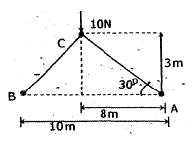Solution: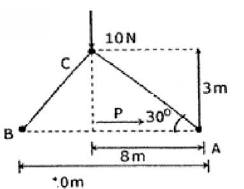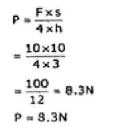QUESTION: 7

What is the ratio of radius of gyrations of two bars of small diameters, in first case axis is at end and in other case axis at centre of bar?

Solution: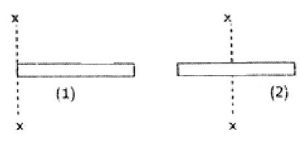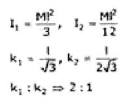QUESTION: 8

A 50 kg block is pulled up on 30° inclined plane by a constant force 800 N. Co-efficient of friction between block and plane is 0.5. What is the velocity of block after 10 seconds?

Solution: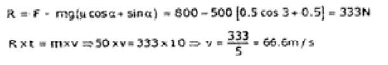QUESTION: 9

For a hexagonal cross sectional bar as per designer specification held in such a way that the total bar is having line contact with ground, but manufacturer placed that bar in such a way that it has area contact with ground. In which case it has more strength?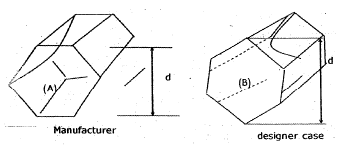Solution: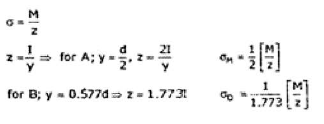QUESTION: 10

A differential pulley is used to lift a weight of 200 N as shown in figure. What is the minimum force required to lift the weight? Take smaller pulley radius as 2 m and larger pulley radius as 5 m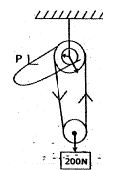Solution: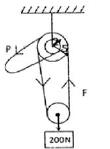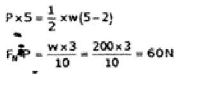*Answer can only contain numeric values
QUESTION: 11

For an equilateral triangle ABC, center of gravity is at the centre of circle which is inscribed in a triangle drawn at the midpoints of side of triangle. The distance of centre of gravity from side AB is _________ m.

Solution: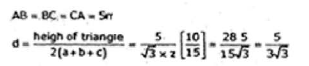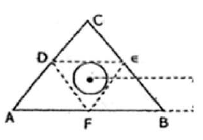QUESTION: 12

A solid pyramid height is 10m. Find out the centre of gravity distance from the base of pyramid.

Solution:

a = h/4 = 10/4 = 2.5 m

QUESTION: 13

A ladder rests against a frictionless wall. The coefficient of friction between ladder and the ground is 0.8. What is the smallest angle θ at which the ladder will remain stationary?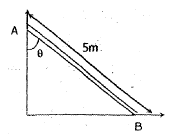Solution: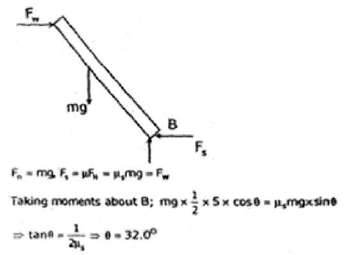QUESTION: 14

A person of weight 60 kg and carrying a bag weight of 20 kg. He tries to climb wall where ladder of mass 200 kg is placed, co-efficient of reaction between wall and ladder is 0.8 and ladder and ground is 0.9. When he is at 3/5 of the way up the ladder, it starts to slip. What was the angle between ladder and land when he starts climbing?

Solution: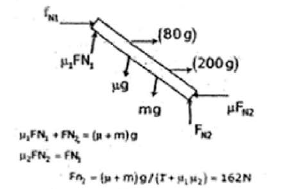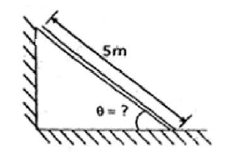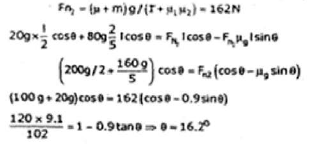QUESTION: 15

A car moving with 96 kmph decides to overtake a truck which is moving with 96 kmph. So car accelerates the speed up to 120 kmph and immediately starts to decelerate until its speed again dropped to 96 kmph. The whole process takes 30 seconds, if the gap between car and truck was 35 m and truck is 10 m long while car is 4m long. What will be the gap between car and track after 30 seconds?

Solution: